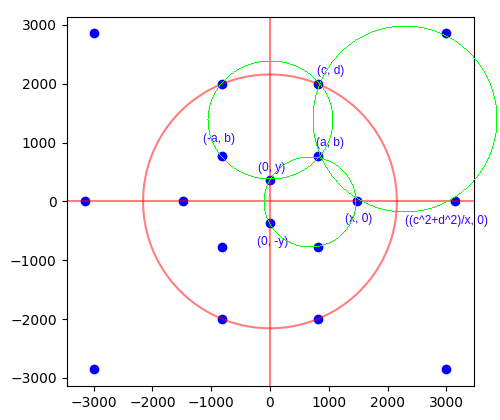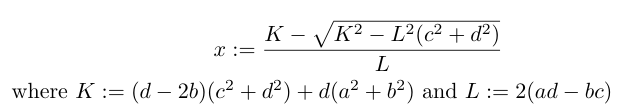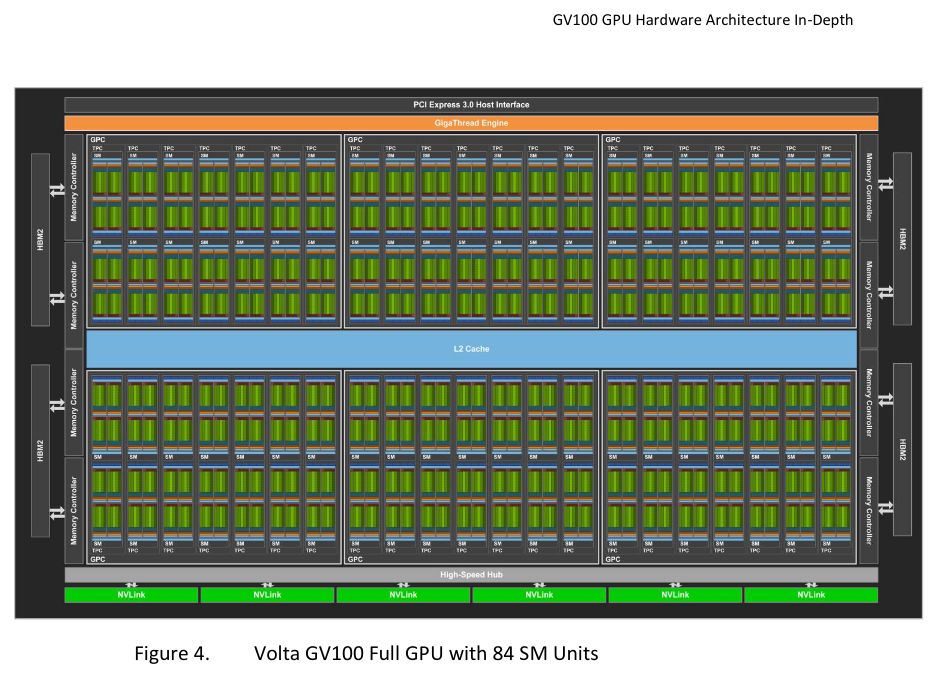# Another two rational dodecahedra

Since finding one rational dodecahedron inscribed in the unit sphere, I decided to port the search program to CUDA so that it can run on a GPU and thereby search a larger space in a reasonable amount of time. Firstly, let us recall our search methodology:We exhaustively look for all small positive integer points (a, b) and (c, d) satisfying b < d and ad > bc. The first of these inequalities ensures that (c, d) is further up the upper-left circle than (a, b); the second inequality ensures that the line through the origin and (a, b) intersects the upper-right circle at two distinct points.

For each such candidate pair of points, we analytically compute x and y, checking that they are rational. If so, we check that the four indicated points on the lower circle are indeed concyclic by performing a final determinant check.

This is embarrassingly parallel, so well-suited for a GPU. We launch one thread for each pair of 1 ≤ a, dN, where N = 10000, so a total of 10^8 threads are launched. Each thread performs two nested loops: the outer loop searches each value of b up to (but excluding) d; the inner loop starts with c = 1 and increments it until the inequality ad > bc is violated or c exceeds the bound N.

### Dealing with integer overflow

One rather annoying bugbear for searching a larger space was that of integer overflow. In the process of computing x, we need to take the square-root of a degree-6 polynomial in the four integer parameters a, b, c, d and check that this square-root is an integer*:*since the expression for x is rational if and only if$\sqrt{K^2 - L^2(c^2 + d^2)}$ is an integer.

When the integer parameters grow beyond 1000, this degree-6 polynomial can easily overflow the maximum representable 64-bit integer. To circumvent this problem, we take the following ugly approach:

• Compute$K^2 - L^2(c^2 + d^2)$ in both double-precision floating-point arithmetic (which is approximate) and in 64-bit integer arithmetic (which is exact, but reduced modulo 2^64).
• ‘Repair’ any loss of relative accuracy (caused by the subtraction) in the double-precision approximation by rounding to the nearest multiple of 2^64 and adding the exact result reduced into the interval [-2^63, 2^63 – 1].
• Compute the double-precision square-root. This will have comparable relative error, and therefore small absolute error (i.e. less than 1).
• Cast the square-root back to a 64-bit integer (which won’t overflow).
• Check this integer and nearby values to see whether they square (modulo 2^64) to the desired result.

Source code: here.

The analogous computation for y involves polynomials of degree at most 4, so we can safely search all a, b, c, d <= 10000 without overflow. The final determinant check is just the evaluation of a polynomial, so we can use arithmetic modulo 2^64 and not worry about integer overflow until later.

### Hardware

I opted to use an NVIDIA Volta V100 because it’s the most powerful GPU to which I currently have access (via Amazon Web Services). The V100 is huge, consisting of 80 streaming multiprocessors, each of which is capable of simultaneously issuing 4 instructions per cycle, where each instruction is vectorised over 32 ‘threads’. (For this reason, the GPU is sometimes advertised as having 80 × 4 × 32 = 5120 ‘CUDA cores’, but these are not comparable with CPU cores; a modern CPU core with vectorisation capabilities and multithreading is more akin to an entire streaming multiprocessor.)Schematic of the Volta V100 from the whitepaper. Only 80 of the 84 streaming multiprocessors are actually ‘activated’; this means that the fabrication of the chip is allowed to contain up to 4 manufacturing defects without affecting the validity of the resulting GPU.

Each of the 80 streaming multiprocessors also has 8 ‘tensor cores’, which perform reduced-precision matrix multiplications useful for neural networks. These tensor cores were unused by this search program, as the nature of this particular problem requires high-precision 64-bit integer and double-precision floating-point computations.

Compiling the program with the switch -Xptxas=-v shows that there is no expensive register spillage or other performance problems:

$nvcc -O3 -Xptxas=-v -lineinfo cudodeca.cu -o cudodeca ptxas info : 56 bytes gmem ptxas info : Compiling entry function '_Z12dodecakernelv' for 'sm_52' ptxas info : Function properties for _Z12dodecakernelv 64 bytes stack frame, 0 bytes spill stores, 0 bytes spill loads ptxas info : Used 47 registers, 320 bytes cmem, 24 bytes cmem Sixteen hours of continual uninterrupted runtime on the V100 (costing a total of about fifty US dollars) were sufficient to exhaust this space and show that there exist at most 3 primitive solutions within these bounds (full output here). The second and third solutions are much larger than the first: Solution 1: a=22, b=21, c=22, d=54, x=40, y=10 Solution 2: a=1680, b=1474, c=2023, d=2601, x=2890, y=578 Solution 3: a=2860, b=3915, c=3297, d=6594, x=7065, y=2355  The reason for saying ‘at most 3 primitive solutions’ is that the final determinant check is performed modulo 2^64, so false positives can slip through. To be absolutely sure of these results, we need an additional manual verification step using unbounded (‘bigint’) integer arithmetic. Unbounded integers are natively provided in languages such as Python, Haskell, and Wolfram Mathematica; we use the latter for convenience. ### Verification We can check the three determinants for the putative solution (1680, 1474, 2023, 2601, 2890, 578)… $ wolfram12.0.0
Mathematica 12.0.0 Kernel for Linux x86 (64-bit)

In:= f = Function[{x, y}, {1, x, y, x^2 + y^2}]

Out= Function[{x, y}, {1, x, y, x^2 + y^2}]

In:= Det[{f[a, b], f[x, 0], f[0, y], f[0, -y]}] /. {a -> 1680, b -> 1474, c -> 2023, d -> 2601, x -> 2890, y -> 578}

Out= 0

In:= Det[{f[(c^2+d^2)/x, 0], f[c, d], f[a, b], f[x, 0]}] /. {a -> 1680, b -> 1474, c -> 2023, d -> 2601, x -> 2890, y -> 578}

Out= 0

In:= Det[{f[c, d], f[a, b], f[-a, b], f[0, y]}] /. {a -> 1680, b -> 1474, c -> 2023, d -> 2601, x -> 2890, y -> 578}

Out= 0

…and likewise for the putative solution (2860, 3915, 3297, 6594, 7065, 2355)…

In:= Det[{f[a, b], f[x, 0], f[0, y], f[0, -y]}] /. {a -> 2860, b -> 3915, c -> 3297, d -> 6594, x -> 7065, y -> 2355}

Out= 0

In:= Det[{f[c, d], f[a, b], f[-a, b], f[0, y]}] /. {a -> 2860, b -> 3915, c -> 3297, d -> 6594, x -> 7065, y -> 2355}

Out= 0

In:= Det[{f[(c^2+d^2)/x, 0], f[c, d], f[a, b], f[x, 0]}] /. {a -> 2860, b -> 3915, c -> 3297, d -> 6594, x -> 7065, y -> 2355}

Out= 0

…confirming that these are indeed valid primitive solutions.

This entry was posted in Uncategorized. Bookmark the permalink.

### 14 Responses to Another two rational dodecahedra

1.Anonymous says:

It turns out that x and y can be written as a rational expression in terms of a, b, c, d (albeit with unwieldy and extremely high degree terms!) by eliminating the terms from the three equations. You then end up with a single homogeneous degree 30 equation in a, b, c, and d. Unfortunately, the algebra system I am using is currently crashing trying to figure out what that equation is. I’m hoping that factors drop out that reduce the degree and allow for an easier solution. Hopefully this will allow us to find an infinite family of solutions.

•Anonymous says:

Actually, more like degree 22 after removing easy factors. But still, it’s a ridiculous equation.

•apgoucher says:

Interesting! Did you make any further progress on simplifying the equation without causing the computer algebra system to crash?

2.Thomas says:

I began thinking about this question again recently, and I realized something… the point (a,b) (and it’s orbit under the symmetry group) is irrelevant, except that it is a common intersection point of three circles.

It should therefore be possible to rewrite the three defining equations as one equation which forces the three circles defined by the points:

Circle A: (0,y), (0,-y), (x,0)
Circle B: (0,y), (c,d), (-c,d)
Circle C: (x,0), ((c^2+d^2)/x, 0), (c,d)

To intersect at one common point (namely, (a,b)). Is there some larger determinant where given six points in the above configuration, is zero when the circles intersect at one common point? This would seem to be the most elegant way to work out the equation.

From a brute force point of view, it should be fairly simple to work out (a,b) as an algebraic fraction in terms of x, y, c, and d based on it being the intersection of circles A and B (that isn’t (0,y)). Then using the concyclicity formula for circle C, we should arrive at one equation. I don’t have direct access to paper to work this out right now, but I will later tonight.

•tomtom2357 says:

So, having calculated the rational expressions for a and b in terms of c, d, x, and y, it turns out they are a degree 7 term over a degree 6 term (the same denominator for both). If you plug this into the determinant equation of the final circle, this would (I haven’t done those calculations yet), result in a degree 16 equation in c, d, x, and y. This still seems awfully huge, so perhaps there is some further simplification I haven’t thought of so far.

I have graphed my progress on desmos, so as to make absolutely certain there are no mistakes in my calculations (it has caught many mistakes). Here is the current version https://www.desmos.com/calculator/lgf9vbfspw. (x,0) is renamed (f,0), and (0,y) is renamed (0,g) to not confuse the software. You can move those points and (c,d) around and see what happens. (a,b) will automatically move around to work with the transformation.

Playing around a bit, there seem to be some degeneracies that may result in extra factors dropping out. For example, you can move the point (x,0) so that the points (a,b) and (c,d) coincide, or so that x^2=c^2+d^2.

I still feel like I’m bashing the problem with a giant stick rather than finding the elegant solution. I really hope there is some sort of determinant formulation that will make this whole situation make more sense. For now though, I will continue to slog through the long way around.

•tomtom2357 says:

So it turns out that there is a way to figure this out, using the Cayley-Menger determinant, once you have the centers and radii of the three circles. https://mathoverflow.net/questions/424583/formula-for-cointersection-of-three-circles is where I have been asking the questions around that. The links from Gro-Tsen are particularly good. He has computed the condition for a set of nine points (3×3) to describe three circles which intersect. It is a degree 18 polynomial in 18 variables, which unfortunately doesn’t seem to factorize.

Where it gets interesting is his second calculation. When you make the 3×3 matrix of coordinates symmetric, this reduces the number of coordinates to six, three of which (the off diagonal terms) are the pairwise intersections of the circles. This time, the degree 18 polynomial splits into three degree 4 factors and a degree 6 factor. The three degree 4 factors I believe correspond to when the common intersection of the three circles is at one of the given coordinates.

The degree 6 term therefore, must represent when the common intersection point is a new point. It has exactly 720 terms, 360 of which are negative. The fact that it is exactly 6! makes me think it must be a determinant of some 6×6 matrix, where each entry in the matrix is one of the 12 variables. I have no idea how to find this matrix though (if it even exists).

Back to the dodecahedron problem: I have found the equation! Here it is:
xy(cy+dx)+(c^2+d^2)(cx+dy-x^2-y^2)=0. I have tested it on all three solutions. Amazingly, the degree is only 4. This means it should be amenable to number theoretic methods. I’ll give this a go next.

•apgoucher says:

That’s huge progress — well done! Quick question: your polynomial is equivalent to the three circles intersecting at a common point, but how do we know that this intersection point (a, b) is a rational point?

Oh, I think that I see it now: the equations for the circles have rational coefficients, and if you take a pair of those circles and solve for the two intersection points, you get (for each coordinate) a quadratic equation with rational coefficients. But we know that one of the two intersection points is rational, so the other intersection point must also be rational.

So it looks like you’ve indeed reduced this problem to finding rational points on a (projective) quartic surface.

Hmm… if we fix c and d, or fix x and y, then we get a cubic equation (probably an elliptic curve!) in the remaining two variables. So it seems like you can just choose one of my three brute-force solutions, set c and d accordingly, and then take multiples (under the usual group law) of that point on the resulting elliptic curve. That should give an infinite family, should it not?

(More easily, just take the power-of-(-2)-indexed multiples of that point in the following manner: define$P_0$ to be the initial solution, and$P_{n+1}$ to be the intersection of the tangent line at$P_n$ with the elliptic curve.)

•apgoucher says:

In fact… let’s fix$x = 4$ and$y = 1$. Then we get the nice simple equation:$c^2 d+4 c^3-17 c^2+4 c d^2+4 c+d^3-17 d^2+16 d = 0$

The first rational dodecahedron gives us an initial rational solution, namely$(c, d) = (11/5, 27/5)$, and we should be able to use that to generate a bunch of further solutions (unless I’m terribly unlucky and chose a torsion point).

•apgoucher says:

Yes, the next solution is$(c, d) = (705/272, 195/68)$.

3.tomtom2357 says:

Yes, the point (a,b) is rational for exactly the reason you said. It is the solution of a quadratic equation, but the other intersection point is given, so it will be rational in the other coordinates. The equation, as you said, is an elliptic curve in (x,y), or in (c,d). We can actually do better than just using the three points you found. We can use the trivial points, where (c,d) = (0,0), (x,0), or (0,y), to construct suitable solutions. Hopefully this can lead to a parametric family of solutions.

On further investigation, it appears to be heinously complicated to get a solution in the right region, so that none of the circles intersect at points they are not supposed to. It will work eventually, but the degree might be quite large

•apgoucher says:

Don’t we already have an infinite family of solutions, namely the points on the elliptic curve where we fix$(x, y) = (4, 1)$? Is there some reason you don’t consider this to be a parametric family? The multiples of a point can be described by a closed form involving the Weierstrass P-function, I think.

•tomtom2357 says:

Yes it is an infinite family. I guess what I was looking for is a polynomial identity where every coordinate is represented by a polynomial in one or two parameters, especially one where (c,d) or (x,y) can be chosen arbitrarily

•apgoucher says:

Done! I used your approach of starting with a trivial solution, but I opted for a slightly different trivial solution (the point at infinity) and only needed to double the point twice to find something that is in the correct region (all variables positive, and with$x, y \ll \sqrt{c^2 + d^2}$ ) for many parameter choices of c and d.

https://cp4space.hatsya.com/2022/06/20/infinitely-many-rational-dodecahedra/